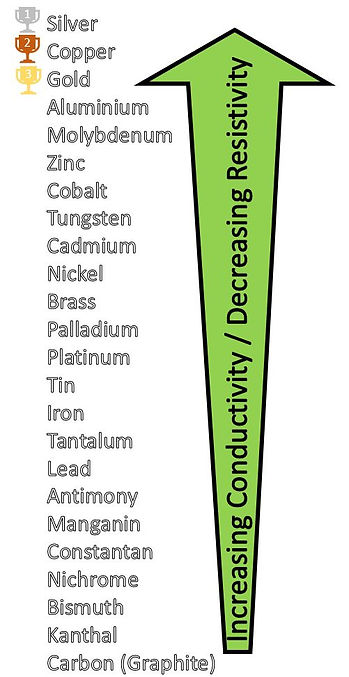top of page

Dividing both sides of the equation by L, we get

Voltage Drop Calculator

Potential difference (Voltage) that exists across an electrical transmission cable or wire is known as Voltage Drop. The voltage across the load toward the end of the transmission cable shall be lower than the supply voltage by a value equals to the voltage drop across the transmission cable.

V Load = V Supply – V DropA simple example that illustrate the calculation of voltage drop of a single-phase supply across a 1.2 km copper conductor is as shown below.Voltage Drop Formula

The formula that governs voltage drop is the Ohm’s Law

Voltage Drop, V = I R

Where

V         = Voltage Drop or potential difference across the conductor (V)

I           = Load current or current that flows across the conductor (A)

R         = Total Resistance across the length of conductor (Ohm)

Resistance per Unit Length, R/L

To determine R, which is the total resistance across the whole length of a conductor, we can first derive the Resistance per unit length of a conductor, R/L based on its material and size (cross-sectional area).To determine R/L, we need to determine the Resistivity, ρ and the Cross-sectional area, A of the Conductor.

Resistivity, ρ

Resistivity, ρ of different types of conductors (at 20°C) can be referred from the tabulation below. Since Resistivity, ρ is the inverse of Conductivity, σ, the most Conductive Material shall have the lowest value of Resistivity. From the tabulation below, it is shown that Pure Silver is the most conductive material, followed by Copper, Gold and Aluminium.Cross-Sectional Area, A

Cables can exist in a variety of cross-sectional areas. The commonly used cable sizes according to the Standardised American Wire Gauge (AWG) system has the following diameters and cross-sectional areas, A.With Resistivity, ρ and Cross-sectional Area, A, Resistance per unit Length, R/L for the specific conductor can be computed as shown in the example below, for 4/0 Gauge 0000 AWG Copper Cable:All values of R/L until AWG 40 can be computed using the same formula as shown. Incorporating the formula into an Excel (Spreadsheet) enables the computation of the R/L for all types of Conductors at all conductor sizes to be done by just selecting the types of Conductors in a Drop-Down List (Please refer to Downloadable Excel File Attached below). Download the Excel File to discover all formulae that have been incorporated into the computation of Voltage Drop in this Calculator.

Voltage Drop Calculator (Excel)Voltage Drop

Last but not least, with the value of R/L, voltage drop can be computed based on the following equations for three phase and single-phase (or DC) systems. This is shown in the example at the very beginning of this page.

The value of Voltage Drop shall be divided the number of conductors if there are extra sets of conductors actively transmitting electricity. This is because the total conductor Cross-Sectional Area, A doubles up when 2 sets of conductors are in use. Hence the value of Resistance per Unit Length, R/L shall be divided by a doubled up Area when 2 sets of conductors are in use.The generally accepted percentage voltage drop is < 4% of the supply voltage in order that the final voltage level across the load does not deviate more than an acceptable threshold from the supply voltage. These parameters shall be computed using the equations as shown below.bottom of page